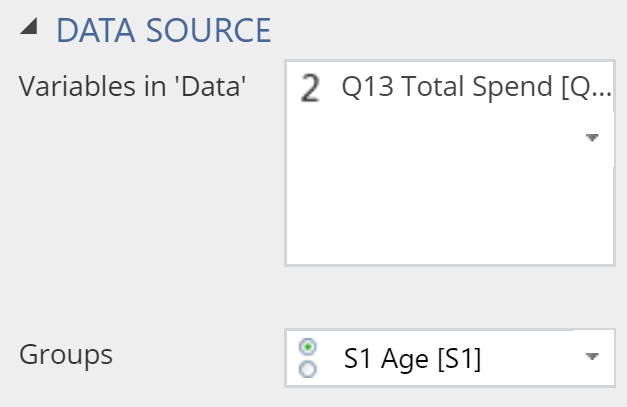# Visualization - Distributions - Bean

A Bean Plot is a mirrored density plot, with a rug plot between the densities showing unique values. Similar to a boxplot and histogram, bean plots are used to compare the distributions of different groups.

## Example

The example below uses data from a fast-food tracking study. The bean plot shows the distribution of fast food consumed for different age groups.

### Create a Bean Plot in Displayr

1. Go to Insert > Visualization > Bean Plot
2. Under Inputs > DATA SOURCE > Variables in ‘Data’, select the variable you want to examine
3. Under Inputs > DATA SOURCE > Groups, select the variable to split your data## Object Inspector Options

The following is an explanation of the options available in the Object Inspector for this specific visualization. Refer to Visualization Options for general chart formatting options.

### Chart

APPEARANCE
Plot vertically Rotates the bean plot by 90 degrees.
Data value color Sets the value of the dashes in the rug.
Bandwidth The relative width of the bandwidth used to compute the density (the smaller the value, the 'bumpier' the plot).
Automatically compute lower bound If selected, the left-side of the plot is set to the lowest value observed in the other data. Otherwise, it is set to be further to the left than this, so as to create a smooth end to the plot.

## Acknowledgements

The density is computed using the base R density function and the plot is created using plotly.

## Code

{
"formChartType": "Bean",
"formStackSeries": false,
"formSmallMultiples": false,
"formAsPercentages": false,
"formScatterLabelType": "As hover text"
}